Olympiad Test: Electricity And Circuits - 1

# Olympiad Test: Electricity And Circuits - 1

Test Description

## 20 Questions MCQ Test Science Olympiad Class 6 | Olympiad Test: Electricity And Circuits - 1

Olympiad Test: Electricity And Circuits - 1 for Class 6 2023 is part of Science Olympiad Class 6 preparation. The Olympiad Test: Electricity And Circuits - 1 questions and answers have been prepared according to the Class 6 exam syllabus.The Olympiad Test: Electricity And Circuits - 1 MCQs are made for Class 6 2023 Exam. Find important definitions, questions, notes, meanings, examples, exercises, MCQs and online tests for Olympiad Test: Electricity And Circuits - 1 below.
 1 Crore+ students have signed up on EduRev. Have you?
Olympiad Test: Electricity And Circuits - 1 - Question 1

### Moving an electron within an electric field would change the ___________the electron.

Detailed Solution for Olympiad Test: Electricity And Circuits - 1 - Question 1

When a force is required to move an electron in the direction of an electric field, its electrical potential energy increases. On the other hand, an electron moving opposite the direction of the electric field will decrease its electrical potential energy.

Olympiad Test: Electricity And Circuits - 1 - Question 2

### Which one of the following statements is false?

Olympiad Test: Electricity And Circuits - 1 - Question 3

### The diagram below shows an open circuit.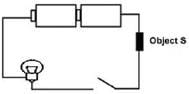When the circuit is closed, the light bulb does not light up at all. Q. What could be the possible reasons? 1. The light bulb has fused. 2. Object S is a plastic ruler. 3. The batteries are too strong. 4. Object S is an insulator of electricity. 5. The arrangement of batteries is correct.

Olympiad Test: Electricity And Circuits - 1 - Question 4

Which of the following does not belong to the group formed by the others?

Detailed Solution for Olympiad Test: Electricity And Circuits - 1 - Question 4

A ceramic is an inorganic non-metallic solid made up of either metal or nonmetal compounds that have been shaped and then hardened by heating to high temperatures. In general, they are hard, corrosion-resistant and brittle.

Olympiad Test: Electricity And Circuits - 1 - Question 5

The filament of a bulb is usually a:

Olympiad Test: Electricity And Circuits - 1 - Question 6

The main function of a switch is to:

Olympiad Test: Electricity And Circuits - 1 - Question 7

Look at the following circuit and read the following paragraph carefully.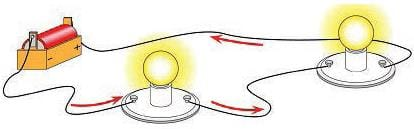All parts are connected one after another. Electrons flow from the negative side of the battery around in a loop to the positive side.

Q. Which of the following statement is related to the circuit described in the paragraph above?

Olympiad Test: Electricity And Circuits - 1 - Question 8

If a light bulb is missing or broken in a parallel circuit, will the other bulb light? Explain.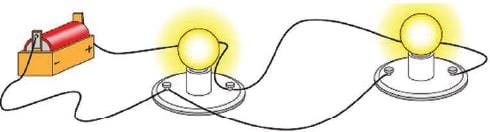Olympiad Test: Electricity And Circuits - 1 - Question 9

Voltage _________ an electrical circuit.

Olympiad Test: Electricity And Circuits - 1 - Question 10

If a battery provides a high voltage, it can __________.

Olympiad Test: Electricity And Circuits - 1 - Question 11

Direction: Alisha has a mobile phone. Energy is stored in the battery of the phone. The diagram below shows the battery being charged.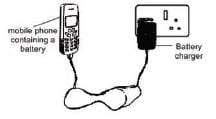Q. The main energy conversion in the battery as it is being charged?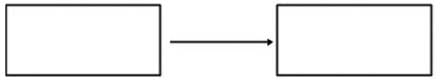Olympiad Test: Electricity And Circuits - 1 - Question 12

Direction: Alisha has a mobile phone. Energy is stored in the battery of the phone. The diagram below shows the battery being charged.Q. When the phone is fully charged, Alisha unplugs the battery charger from the phone. State the energy conversion when the mobile phone rings.

Detailed Solution for Olympiad Test: Electricity And Circuits - 1 - Question 12

Olympiad Test: Electricity And Circuits - 1 - Question 13

Use the following diagram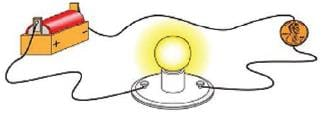Q. What supplies energy in an electric circuit?

Olympiad Test: Electricity And Circuits - 1 - Question 14

Use the following diagramQ. Which material is a conductor?

Olympiad Test: Electricity And Circuits - 1 - Question 15

Which of these could be used as a resistor in a circuit?

Olympiad Test: Electricity And Circuits - 1 - Question 16

Which statement is not correct about current electricity?

Olympiad Test: Electricity And Circuits - 1 - Question 17

In domestic wiring in India, the neutral wire has which of the following colors?

Olympiad Test: Electricity And Circuits - 1 - Question 18

In a circuit having one bulb, another bulb is added. The new bulb will:

Olympiad Test: Electricity And Circuits - 1 - Question 19

Study the circuit diagram below. The bulbs are labelled A, B, C and D. The switches are labeled 1, 2, 3 and 4. Use the diagram to solve question: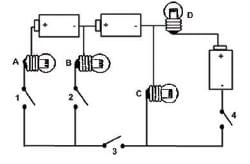Q. Which two switches should be closed so that only bulbs B and C would light up?

Olympiad Test: Electricity And Circuits - 1 - Question 20

Study the circuit diagram below. The bulbs are labelled A, B, C and D. The switches are labeled 1, 2, 3 and 4. Use the diagram to solve question:Q. Which bulb(s) will remain lit if bulb D fuses and all the switches are closed?(Scan QR code)